SSC (English Medium) Class 8Maharashtra State Board
Share

# Draw an Obtuse Angled δ Lmn. Draw Its Altitudes and Denote the Orthocentre by ‘O’. - SSC (English Medium) Class 8 - Mathematics

ConceptMedians of a Triangle

#### Question

Draw an obtuse angled Δ LMN. Draw its altitudes and denote the orthocentre by ‘O’.

#### Solution

To draw an obtuse-angled ∆LMN.

i. Draw a base line of any length, mark it MN. At M draw an obtuse angle mark that line point L. Join L and N points. ΔLMN thus formed is an obtuse angled triangle.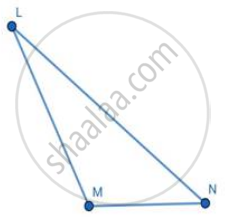ii. To draw an altitude from vertex L, extend side MN of the triangle from point M with a dashed line, as shown in the figure, and then draw the perpendicular lines from M.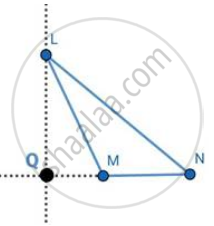iii. Considering side LN as a base, draw an altitude MP on side LN. Seg MP is an altitude on side LN.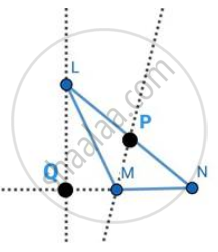iv. To draw altitude from vertex N, extend side LM of the triangle from point M with dashed line, as shown in the figure, and then draw the perpendicular line from vertex N.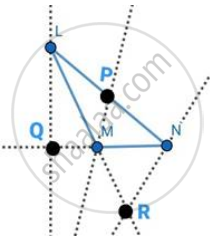v. Now for the orthocentre, as all the altitudes do not intersect we'll have to extend them so that they can meet giving us an orthocentre of the triangle.

vi. Hence, extend the altitude LQ, from point Q ; MP from point M, and NR from point R.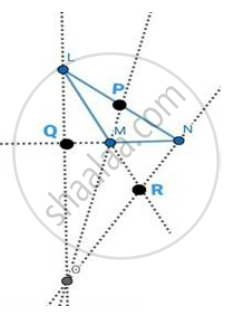vii. The ortho centre of the Obtuse triangle lies outside the triangle.

viii. The point O denotes the orthocentre of the obtuse-angled ∆LMN.

Is there an error in this question or solution?

#### APPEARS IN

Balbharati Solution for Balbharati Class 8 Mathematics (2019 to Current)
Chapter 4: Altitudes and Medians of a triangle
Practice Set 4.1 | Q: 4 | Page no. 22
Solution Draw an Obtuse Angled δ Lmn. Draw Its Altitudes and Denote the Orthocentre by ‘O’. Concept: Medians of a Triangle.
S